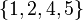# S4 in S5

This article is about a particular subgroup in a group, up to equivalence of subgroups (i.e., an isomorphism of groups that induces the corresponding isomorphism of subgroups). The subgroup is (up to isomorphism) symmetric group:S4 and the group is (up to isomorphism) symmetric group:S5 (see subgroup structure of symmetric group:S5).
VIEW: Group-subgroup pairs with the same subgroup part | Group-subgroup pairs with the same group part | All pages on particular subgroups in groups

## Definition

The group$G$ is taken as symmetric group:S5: the symmetric group of degree five. For concreteness, we take$G$ as the symmetric group on the set$\{ 1,2,3,4,5 \}$.

We take$H = H_5$ as the subgroup fixing$\{ 5 \}$, so$H$ is symmetric group:S4 acting on the set$\{ 1,2,3,4 \}$.$H$ has four other conjugate subgroups, each corresponding to a different fixed point:

•$H_1$ is the subgroup fixing$\{ 1 \}$, and is the symmetric group on the set$\{ 2,3,4,5 \}$.
•$H_2$ is the subgroup fixing$\{ 2 \}$, and is the symmetric group on the set$\{ 1,3,4,5 \}$.
•$H_3$ is the subgroup fixing$\{ 3 \}$, and is the symmetric group on the set$\{ 1,2,4,5 \}$.
•$H_4$ is the subgroup fixing$\{ 4 \}$, and is the symmetric group on the set$\{ 1,2,3,5 \}$.
•$H = H_5$ is the subgroup fixing$\{ 5 \}$, and is the symmetric group on the set$\{ 1,2,3,4 \}$.

## Arithmetic functions

Function Value Explanation
order of group 120
order of subgroup 24
index of subgroup 5
size of conjugacy class of subgroup 5
number of conjugacy classes in automorphism class of subgroup 1
size of automorphism class of subgroup 5

## Subgroup properties

### Other properties

Property Meaning Satisfied? Explanation Comment
Hall subgroup order and index are relatively prime Yes$\{ 2,3 \}$-Hall subgroup. Also, order (24) and index (5) are relatively prime.
p-complement complement of a$p$-Sylow subgroup Yes$p$-complement for$p = 5$

## GAP implementation

The group-subgroup pair can be constructed as follows:

G := SymmetricGroup(5); H := SymmetricGroup(4);